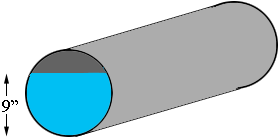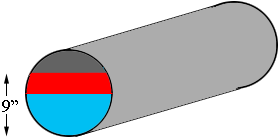SEARCH HOMEMath Central Quandaries & QueriesQuestion from tom, a student: A cylindrical tank, 1 ft in diameter, length 6 ft , is placed so that its axis is horizontal. How many pounds of water will be used in filling it to a depth of 9in. , if water weighs 62.4 lbs. per cu. ft.? does any one know how to illustrate this one? I calculated the weight of the water by getting first the volume through multiplying 9 in (making sure to convert it in foot) and area of the base, yet it doesn't match the real answer (which is 236.56 lbs.)Hi Tom,

I think you missed the fact that the tank is lying on its side, "its axis is horizontal".Penny

Tom replied

but isn't it that " 9 inches" is 3/4 part of the area of the 1ft. diameter base?
then when i calculated for the volume and to pounds....it's still
doesn't match the answer? how is that?

Tom,

I redrew my diagram with a line across the end of the tank half way up and used red to colour the region from 1/2 way up to 3/4 the way up.If your contention is correct then the area of the piece coloured red is equal to the area of the piece coloured gray. But this is certainly not true. They are the same height, 3 inches, but the red part is wider than the gray part.

To see how to calculate the area look at my response to a question from Mario concerning another horizontal cylindrical tank.

PennyMath Central is supported by the University of Regina and The Pacific Institute for the Mathematical Sciences.